Dave's Math Tables: chi2-distribution(Math | Stat | Distributions | chi2-Distributions)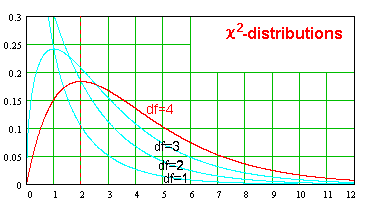The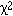-distribution, with n degrees of freedom, is given by the equation:

f() = ()^(n/2 - 1) e^(-/ 2 ) 2^(-n/2) /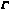(n/2)
The area within an interval (a,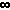) =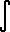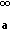f() d=(n/2, a/2) /(n/2) (See also Gamma function)